# Important Questions for Class 11 Chapter 13 - Hydrocarbons.

Class 11 chemistry important questions with answers are provided here for Chapter 13 – Hydrocarbons. These important questions are based on the CBSE board curriculum and correspond to the most recent Class 11 chemistry syllabus. By practising these Class 11 important questions, students will be able to quickly review all of the ideas covered in the chapter and prepare for the Class 11 Annual examinations as well as other entrance exams such as NEET and JEE.

Download Class 11 Chemistry Chapter 13 – Hydrocarbons Important Questions with Answers PDF by clicking on the button below.

## Hydrocarbons Class 11 Chemistry One-Shot## Class 11 Hydrocarbons Important Questions with Answers

Q1. Why do alkenes prefer to undergo electrophilic addition reaction while arenes prefer electrophilic substitution reactions? Explain.

Alkenes are a rich source of loosely held pi (π) electrons, due to which they show electrophilic addition reactions. Significant energy changes accompany electrophilic addition reactions of alkenes, so these are more energetically favourable than electrophilic substitution reactions. In special conditions, alkenes also undergo free radical substitution reactions.

In arenes during electrophilic addition reactions, the aromatic character of the benzene ring is destroyed, while during electrophilic substitution reaction, it remains intact. Electrophilic substitution reactions of arenes are energetically more favourable than electrophilic addition reactions.

That’s why alkenes prefer to undergo electrophilic addition reaction while arenes choose electrophilic substitution reaction.

Q2. Alkynes on reduction with sodium in liquid ammonia form trans alkenes. Will the butene thus formed on reduction of the 2-butyne show the geometrical isomerism?

Yes, butene formed on reducing the 2-butyne will show the geometrical isomerism.

On reduction of but-2-yne, But-2-ene is formed in which both the methyl groups are on the same side or opposite side to show geometrical isomers.

Q3. Rotation around the carbon-carbon single bond of ethane is not completely free. Justify the statement.

Alkanes contain carbon-carbon sigma (σ) bonds. Electron distribution of the sigma molecular orbital is symmetrical around the internuclear axis of the C−C bond, which is not distributed due to rotation about its axis. This permits free rotation about the C−C single bond. This rotation results in different spatial arrangements of atoms in space which can change into one another. Such spatial arrangements of atoms that can be converted into one another by rotation around a C−C single bond are called conformations or conformers or rotamers. Alkanes can have an infinite number of conformations by rotation around C−C single bonds. However, rotation around a C−C single bond is not entirely free. A small energy barrier of 1−20kJmol−1 hinders it due to weak repulsive interaction between the adjacent bonds. Such a type of repulsive interaction is called a torsional strain.

Q4. Draw Newman and Sawhorse projections for the eclipsed and staggered conformations of ethane. Which of these conformations is more stable and why?

The staggered conformation is most stable because the hydrogens and bonding pairs of electrons are at maximum distance, thus causing minimum repulsion. The eclipsed conformation is least stable, and the other intermediate conformation (Gauche or skew form) lies between them.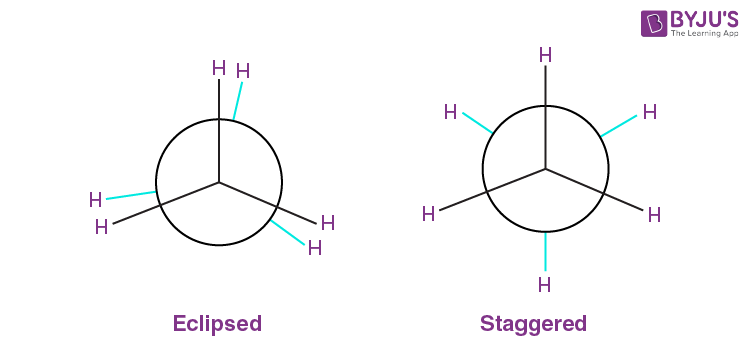Q5. The intermediate carbocation formed in the reaction of HI, HBr and HCl with propene is the same, and the bond energy of HCl, HBr and HI are 430.5 kJmol−1, 363.7 kJmol−1 and 296.8 kJmol−1, respectively. What will be the order of reactivity of these halogen acids?

CH3−CH=CH2 + H+ → CH3−CH+−CH3 + X→ CH3−CHX−CH3

The first step is slow, so it is the rate-determining step. The rate of this step depends on the availability of the proton. This, in turn, depends upon the bond dissociation enthalpy of the H-X molecule.

Lower the bond dissociation enthalpy of the H-X molecule, greater the reactivity of halogen halide. Since the bond dissociation energy decreases in order,

HI < HBr < HCl

Therefore, the reactivity of the halogen acids decreases from HI to HCl. i.e. , HI > HBr > HCl

Q6. What will be the product obtained from the following reaction, and why?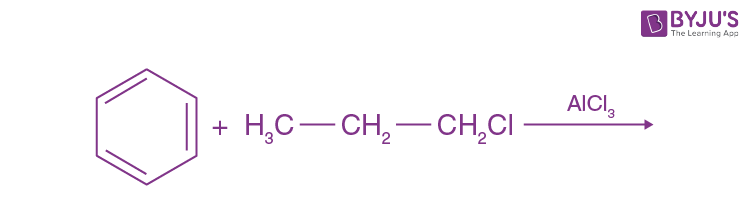Isopropyl Benzene would be formed.

Mechanism:

When Friedel-Crafts alkylation takes place with a higher alkyl halide, primary carbocation is formed first, which converts into secondary carbocation by rearrangement, as it is more stable.

CH3−CH2−CH2Cl + AlCl3 → AlCl4 + CH3−CH2−CH2+ (primary carbocation)

CH3−CH2−CH2+ → CH3+CH-CH3 (Secondary carbocation)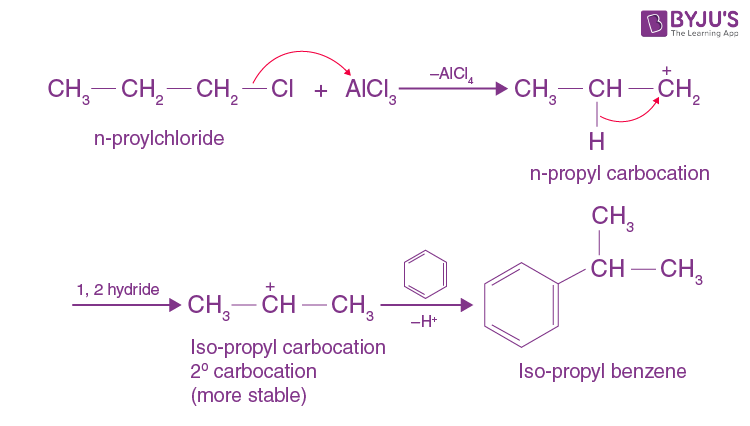Q7. How will you convert benzene into

(i) p – nitrobromobenzene

(ii) m – nitrochlorobenzene

(i) Benzene can be converted into p-nitrobromobenzene as

Electrophilic aromatic substitution of benzene with bromine in ferric bromide gives bromobenzene. Nitration with conc. Nitric acid and concentric sulphuric acid give a mixture of ortho-bromonitrobenzene and para-bromonitrobenzene, separated by fractional distillation.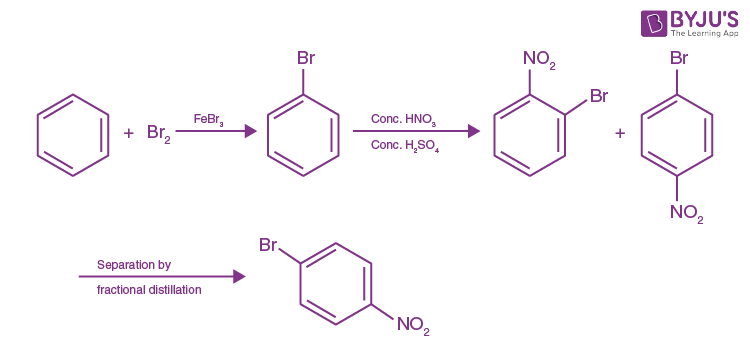(ii) Benzene can be converted into m-nitrochlorobenzene as

Nitration of benzene with conc nitric acid, and conc sulphuric acid gives nitrobenzene. Chlorination with chlorine in anhydrous aluminium chloride gives meta nitro chlorobenzene.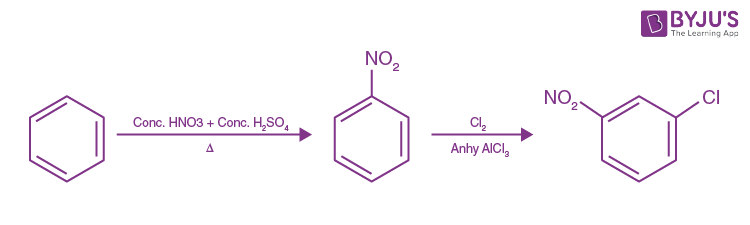Q8. Arrange the following set of compounds in the order of their decreasing relative reactivity with an electrophile. Give reason.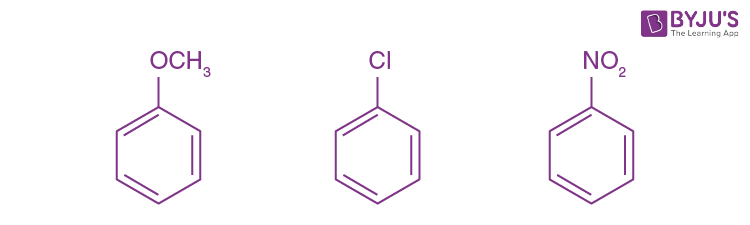The methoxy group (-OCH3) is the electron releasing group. It increases the electron density in the benzene nucleus due to the resonance effect +R-effect. Hence, it makes anisole more reactive than benzene towards the electrophile. In the case of alkyl halides, the electron density increases at ortho and para positions due to the +R effect. However, the halogen atom also withdraws electrons from the ring because of its -I effect. Since the -I effect is stronger than the +R effect, the halogens are moderately deactivating. Thus, the overall electron density on the benzene ring decreases, making further substitution difficult. The -NO2 group is the electron-withdrawing group. It reduces the electron density on the benzene nucleus due to its strong -R-effect and strong -I-effect. Hence, it makes nitrobenzene less.

Therefore, the overall reactivity of these three compounds towards electrophiles decreases in the following order- Anisole (1) > Chlorobenzene (2) > Nitrobenzene(3).

Q9. Despite their – I effect, halogens are o- and p-directing in haloarenes. Explain.

Halogens on benzene rings have a -I and a +R effect. The -I effect deactivates the ring, but the +R effect increases the electron density on ortho and para positions. Hence, halogens are ortho and para directing.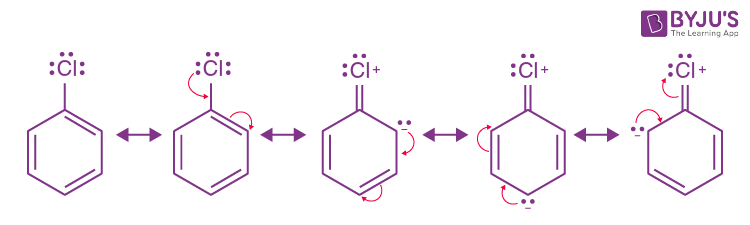Q10. Why does the presence of a nitro group make the benzene ring less reactive than the unsubstituted benzene ring? Explain.

The Nitro group is an electron-withdrawing group (-R and -I effects). It deactivates the ring by decreasing nucleophilicity for further substitution.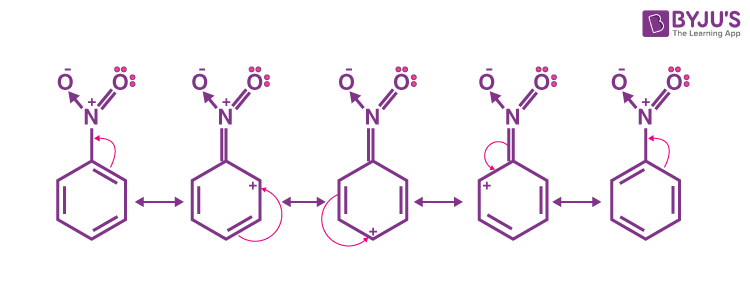Q11. Suggest a route for the preparation of nitrobenzene starting from acetylene?

When passed through a red hot iron tube at 500°C, Acetylene undergoes cyclic polymerisation to give benzene which, upon nitration, gives nitrobenzene.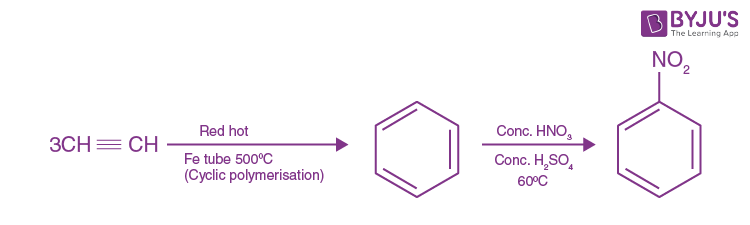Q12. Predict the major product (s) of the following reactions and explain their formation.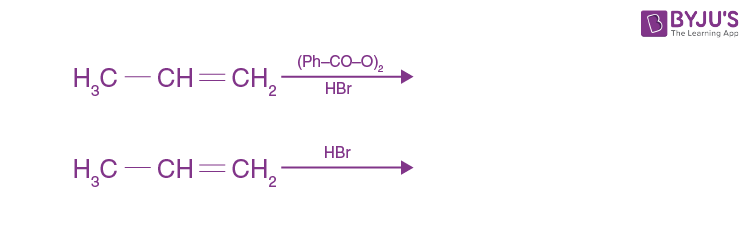(i) H3C−CH2−CH2Br

(ii) H3C−CHBr−CH3

Q13. Nucleophiles and electrophiles are reaction intermediates having electron rich and electron deficient centres respectively. Hence, they tend to attack electron deficient and electron rich centres respectively. Classify the following species as electrophiles and nucleophiles.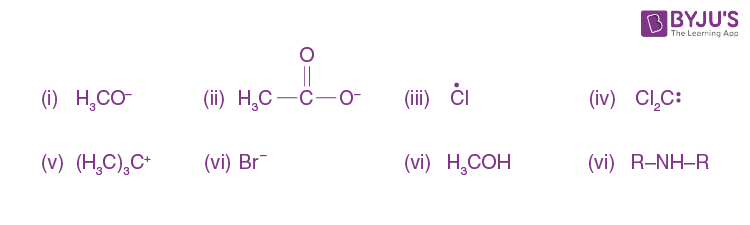Electrophiles are electron seeking species or electron-deficient species. These species may be neutral or positively charged.

(iii) Cl˙ (iv) Cl2C: (v) (H3C)3C+ are electrophiles.

Nucleophiles are electron-rich species and may be neutral or negatively charged.

(i) H3CO (ii) H3COO(iv) Br (vi) CH3OH (viii) R−NH−R are nucleophiles .

Q14. The relative reactivity of 1°, 2°, 3° hydrogen’s towards chlorination is 1: 3.8: 5.

Calculate the percentages of all monochlorinated products obtained from 2-methylbutane.

The possible monochlorinated products obtained from 2-methyl butane are:

• CH2Cl−CH−CH3−CH2−CH3 = (1)
• CH3−CH−CH3−CHCl−CH3 = (2)
• CH3−Cl−C−CH3−CH2−CH3 = (3)

Relative amounts of A, B and C compounds can be calculated as hydrogen multiplied by relative reactivity.

Therefore, The Relative amount of A (1) = 9 × 1 = 9

The relative amount of B (2) = 2 × 3⋅8 = 7.6

The relative amount of C (3) = 5 × 1 = 5

Total amount = 9 + 7⋅6 + 5 = 21⋅6.

Percentage of A =9 / 21.6 × 100 = 41.7%

Percentage of B= 7.6 / 21.6 × 100 = 35.2%

Percentage of C = 5 / 21.6 × 100 = 23.1%

Q15. Write the structures and names of products obtained in the reactions of sodium with a mixture of 1-iodo-2-methylpropane and 2-iodopropane.

Wurtz’s reaction between 1-iodo-2-methylpropane and 2-iodopropane gives the following three products.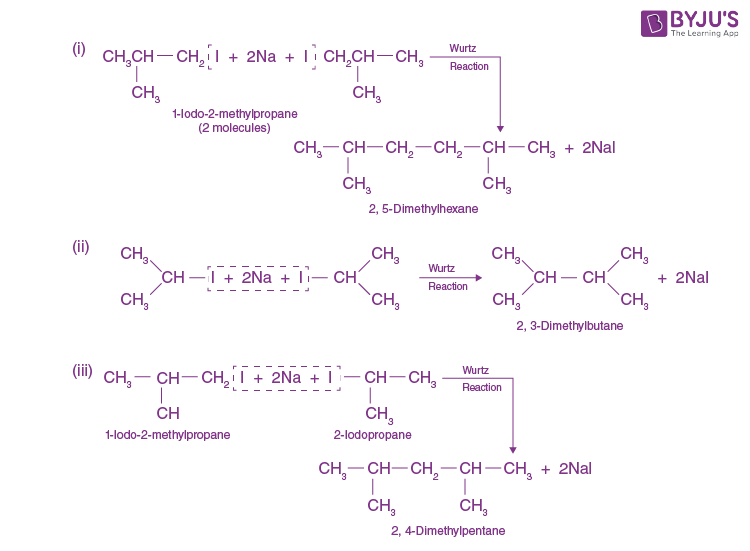Q16. Write hydrocarbon radicals that can be formed as intermediates during monochlorination of 2-methylpropane? Which of them is more stable? Give reasons.

2-methylpropane gives two types of radicals:

• CH3−CH(CH3)−∗CH2
• CH3−∗C(CH3)−CH3

Tertiary 3free radical is more stable because it has 9α hydrogen, and the hyperconjugation structure is stabilised. Primary 1free radical is less stable because of only 1α hydrogen and one hyper conjugative structure.

Hence (2) is more stable than (1)

Q17. An alkane C8H18 is obtained as the only product subjecting a primary alkyl halide to the Wurtz reaction. On monobromination, this alkane yields a single isomer of a tertiary bromide. Write the structure of alkane and the tertiary bromide.

Since the alkane upon monobromination forms a single isomeric tertiary alkyl bromide, the alkane is a symmetrical secondary alkane. The primary alkyl halids, which gives this alkane by Wurtz reaction, is a branched-chain derivation with four carbon atoms. The reactions involved are given as follows.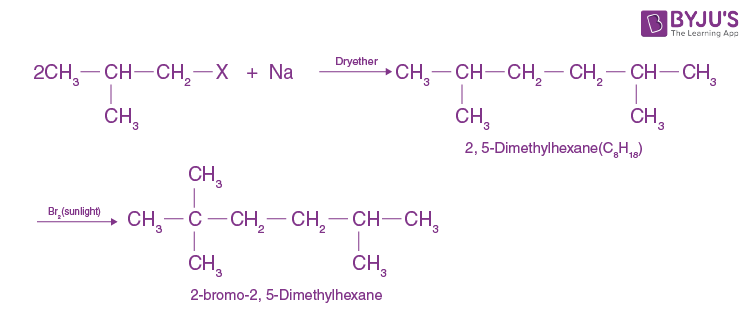Q18. The ring systems having the following characteristics are aromatic.

(i) Planar ring containing conjugated n bonds.

(ii) Complete delocalisation of the n-electrons in the ring system, i.e. each atom in the ring

has an unhybridised n-orbital. and

(ii) Complete delocalisation of the n-electrons in the ring system i.e. each atom in the ring

has unhybridized p-orbital, and

(iii) Presence of (4n+2) n-electrons in the ring where n is an integer (n = 0, 1,….) [Huckel rule].

Using this information, classify the following compounds as aromatic/non-aromatic.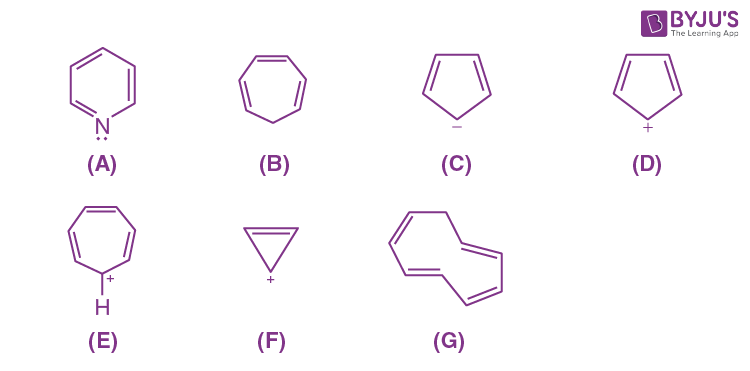A = Planar ring, all atoms of the ring are sp2 hybridised, has six delocalised π electrons, follows Huckel rule. It is aromatic.

B = Has six π electrons, but the delocalisation stops at sp3 hybridised CH2– carbon. Hence, it is not aromatic.

C = Has six delocalised π-electrons (4 π electrons + 2 unshared electrons on negatively charged carbon) in a planar ring, following Huckel’s rule. It is aromatic.

D = Has only four delocalised π-electrons. It is non-aromatic.

E = Has six delocalised π-electrons following Huckel’s rule. π electrons are in sp2 hybridised orbitals, conjugation all over the ring because of positively charged carbon. The ring is planar hence is aromatic.

F = Has 2 π electrons following Huckels Rule i.e. (4n+2) π-electrons where (n=0). It is aromatic.

G = Has 8π electrons do not follow Huckel’s rule, i.e., (4n+2) π-electrons rule. It is not aromatic.

Q19. Which of the following compounds are aromatic according to Huckel’s rule?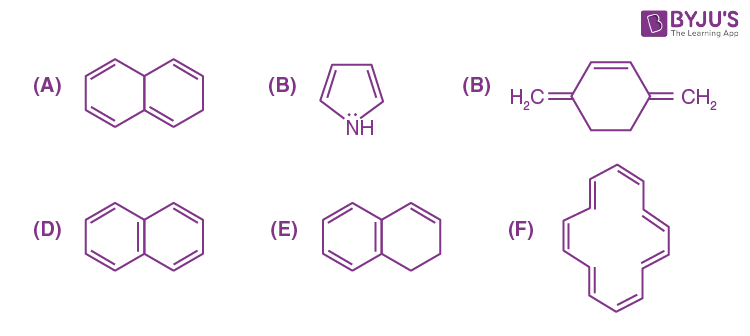A = Has 8π electrons, does not follow Huckel rule. It is not aromatic.

B = Has 6π delocalised electrons. Hence, it is aromatic.

C = Has 6π electrons in conjugation but not in the ring. It is not aromatic. D = Has 10π electrons in planar rings. It is aromatic.

E = Out of 8π electrons, it has delocalised 6π electrons in one six-membered planar ring, thus following Huckel’s rule due to which it will be aromatic.

F = Has 14 π electrons in conjugation. Thus, Huckel’s rule is being followed. The compound will be aromatic if the ring is planar.

Q20. Suggest a route to prepare ethyl hydrogen sulphate (CH3-CH2-OSO2-OH) starting from ethanol (C2H5OH).

We can prepare C2H5OH from CH3-CH2-OSO2-OH in two steps.

Step 1: Protonation of Alcohol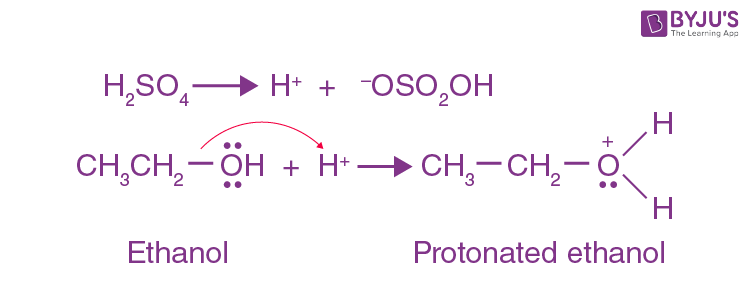Step 2: Attack of Nucleophile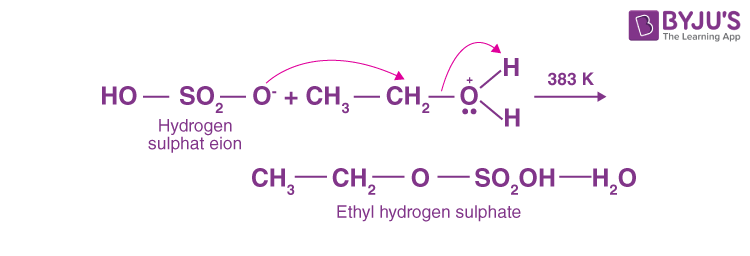The temperature should not rise above 383 K; otherwise, it will produce diethyl ether at 413 K or ethene at 433 K.

Q1. An alkyl halide C5H11Br (A) reacts with ethanolic KOH to give an alkene ‘B’, which reacts with Br2 to give a compound ‘C’, which on dehydrobromination provides an alkyne with ‘D’. On treatment with sodium metal in liquid ammonia, one mole of ‘D’ gives one mole of the sodium salt of ‘D’ and half a mole of hydrogen gas. Complete hydrogenation of ‘D’ yields a straight-chain alkane. Identify A, B, C and D. Give the reactions involved.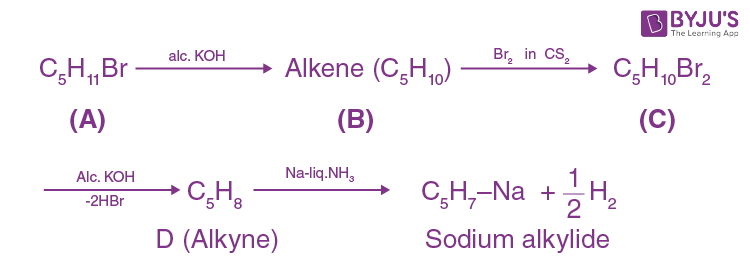The reactions suggest that (D) is a terminal alkyne. This means a triple bond is at the end of the chain. It could be either (I) or (II).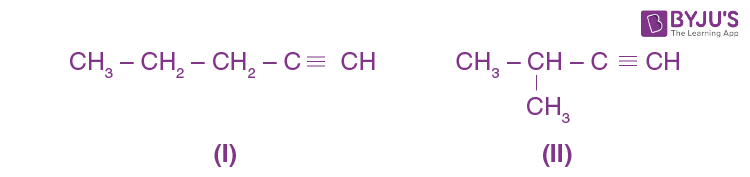Since alkyne ‘D’ on hydrogenation yields straight-chain alkane, the structure I is alkyne (D).

Hence, A, B and C structures are as follows:

1. CH3-CH2-CH2-CH2-CH2Br
2. CH3-CH2-CH2-CH=CH2
3. CH3-CH2-CH2-CH(Br)-CH2Br

Q2. 896 mL vapour of a hydrocarbon ‘A’ having carbon 87.80% and hydrogen 12.19% weighs 3.28g at STP. Hydrogenation of “A’ gives 2-methyl pentane. Also, “A’ on hydration in the presence of H2SO4 and HgSO4 provides a ketone with ‘B’ having molecular formula C6H12O. The ketone ‘B’ gives a positive iodoform test. Find the structure of “A’ and give the reactions involved.

Step 1.

896 ml vapour of CxHy (A) weighs 3.28g

22700 mL vapour of CxHy (A) weighs 3.28 X 22700 / 896 gmol-1

22700 mL vapour of CxHy (A) weighs 83.1 gmol-1

Step 2.

 Element Percentage Atomic Mass Relative Ratio Relative number of atoms Simplest Ratio C 87.8 12 7.31 1 3 H 12.19 1 12.19 1.66 4.98 ⇋ 5

Empirical Formula of ‘A’ C3H5

Empirical Formula mass of ‘A’ = 35 + 5 = 41u

n= Molecular Mass / Empirical Formula mass = 83.1 / 41 = 2.02 = 2

Hence, Molecular mass is double of the empirical formula mass.

Hence, the molecular formula is C6H10

Step 3.

C6H10 + 2H2 → 2- methyl pentane.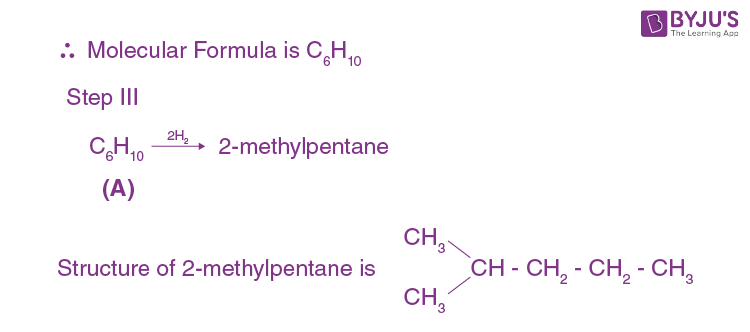Hence, the molecule has a five-carbon chain with a methyl group at the second carbon atom.

‘A’ adds a molecule of H2O in the presence of Hg2+ and H+. It should be an alkyne.

The two possible structures for ‘A’ are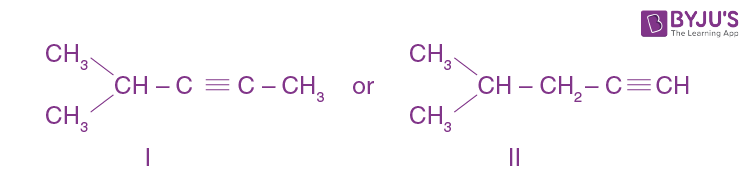Since the ketone (B) gives a positive iodoform test, it should contain a —COCH3 group. Hence the structure of ketone is as follows.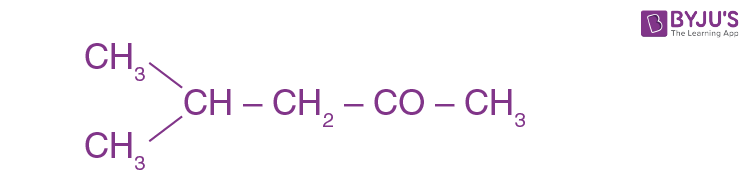Q3. An unsaturated hydrocarbon “A’ adds two molecules of H2 and on reductive ozonolysis gives butane-1,4-di-al, ethanal and propanone. Give the structure of ‘A’, write its IUPAC name and explain the reactions involved.

Two hydrogen molecules add on ‘A’, which shows that ‘A’ is either an alkadiene or an alkyne. On reductive ozonolysis, ‘A’ gives three fragments, one of which is dialdehyde. Hence, the molecule has broken down at two sites. Therefore, ‘A’ has two double bonds. It gives the following three fragments: OHC—CH2—CH2—CHO, CH3CHO and CH3—CO—CH3 Hence, its structure as deduced from the three fragments must be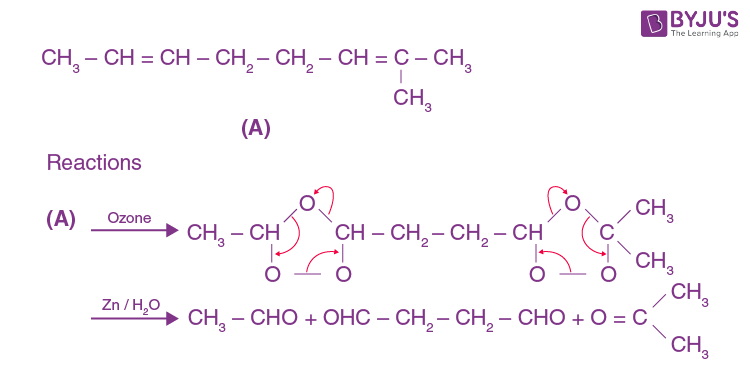Q4. In the presence of peroxide addition of HBr to propene takes place according to anti-markovnikov’s rule but the peroxide effect is not seen in the case of HCI and HI. Explain.